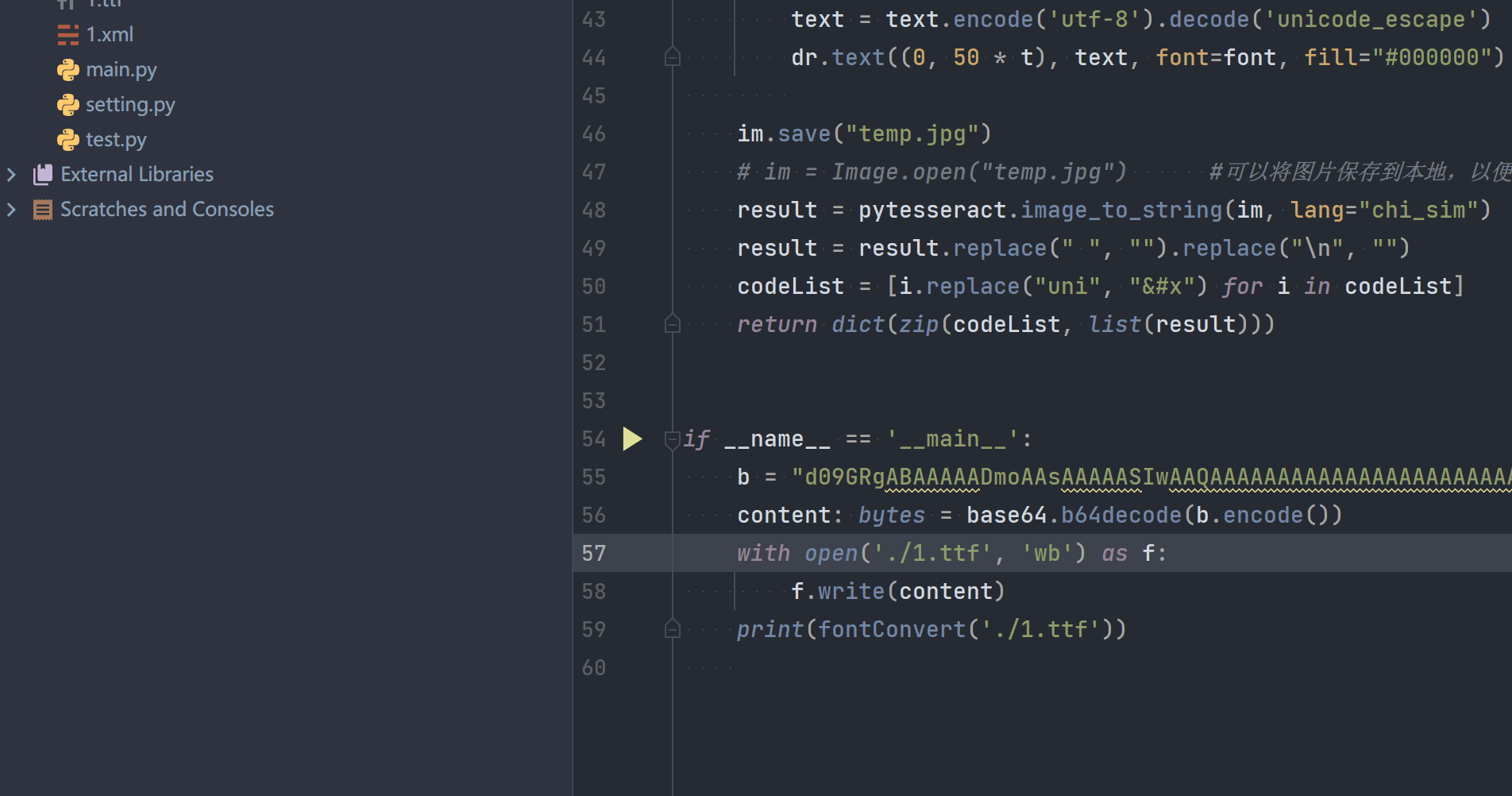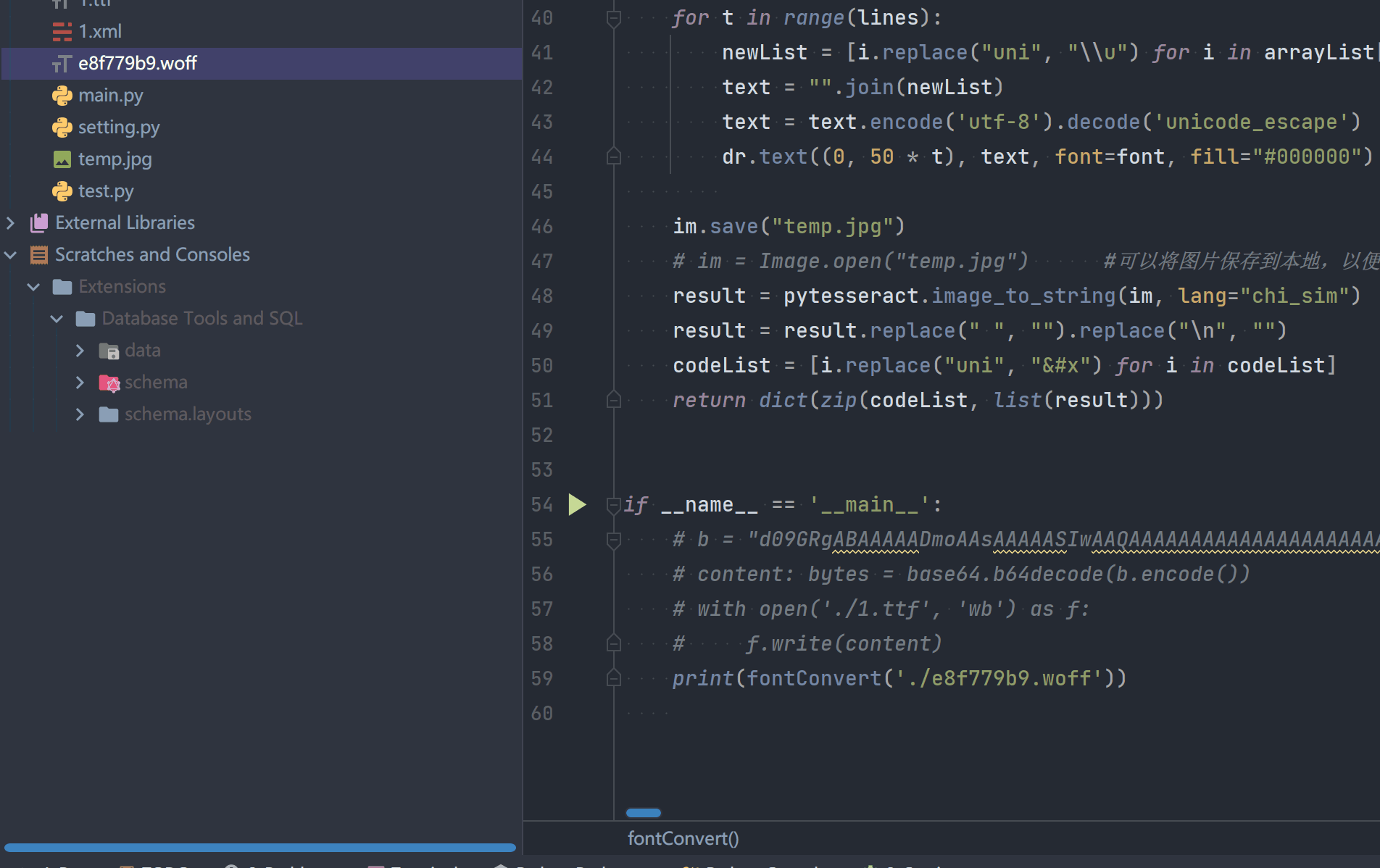# CSS字体加密反反爬通用方法

Posted by mkdir700 on 05-02，2021

## 前言

1. 字体字形坐标点与编码之间的对应关系不会随着多次请求而变化，例如：58同城房子出租
2. 字体字形坐标点每次请求时，位置不固定，但是每个文字的打点数量一致，例如：猿人学第7题
3. 字体的打点坐标点与打点数量随着每次请求都会发生变换，例如：58同城招聘

1. 字体越多，识别花费的时间就越长；
2. 能通过字体文件规律解密的，则不建议使用这种方法；

## 环境配置

### 下载tesseract

tesseract-ocr-setup-3.05.02下载

## 识别代码

``````# -*- coding: utf-8 -*-
"""
Created on 2021/4/27 16:34
---------
@summary:
---------
@author: mkdir700
@email:  mkdir700@gmail.com
"""
import base64

import numpy
import pytesseract
from fontTools.ttLib import TTFont
from PIL import Image, ImageDraw, ImageFont

def fontConvert(fontPath):
"""
解析字体文件
:param fontPath: 字体文件路径
:return: 编码和汉字的对应关系
"""
font = TTFont(fontPath)
codeList = font.getGlyphOrder()[1:]
# print(codeList)

# 创建画布
im = Image.new("RGB", (1800, 1000), (255, 255, 255))
dr = ImageDraw.Draw(im)

# 加载字体文件对象
font = ImageFont.truetype(fontPath, 40)

word_count = len(codeList)
if word_count >= 40:
lines = word_count // 40  # 文字排列的行数，在图片上分行展示
else:
lines = word_count
arrayList = numpy.array_split(codeList, lines)
# print(arrayList)

# 将字体文件中的文字绘制成图片
for t in range(lines):
newList = [i.replace("uni", "\\u") for i in arrayList[t]]
text = "".join(newList)
text = text.encode('utf-8').decode('unicode_escape')
dr.text((0, 50 * t), text, font=font, fill="#000000")

im.save("temp.jpg")
# im = Image.open("temp.jpg")      #可以将图片保存到本地，以便于手动打开图片查看
result = pytesseract.image_to_string(im, lang="chi_sim")
result = result.replace(" ", "").replace("\n", "")
codeList = [i.replace("uni", "&#x") for i in codeList]
return dict(zip(codeList, list(result)))

if __name__ == '__main__':
b = "xxxx="
content: bytes = base64.b64decode(b.encode())
with open('./1.ttf', 'wb') as f:
f.write(content)
print(fontConvert('./1.ttf'))

``````

## 效果演示